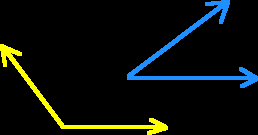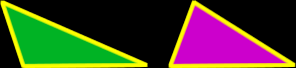# Online Math Dictionary: O

Easy to understand math definitions for K-Algebra mathematics
Just scroll down or click on the word you want and I'll scroll down for you!

 oblique angle oblique prism oblique triangle obtuse angle obtuse triangle octagon octahedron odd numbers order of operations ordered pair origin orthogonal oval

 Oblique AngleAn oblique angle is an angle that is not aright angle.  (An oblique angle is an angle whose measure is not 90 degrees.)Oblique PrismAn oblique prism is a prism that is tilted or slanted a bit.  Technically, an oblique prism is one whosesides do not form 90 degree angles with the bases.Oblique TrianglesAn oblique triangle is a triangle that is not a right triangle.  (An oblique triangle does not have a 90 degree angle.)Obtuse AngleAn obtuse angle is an angle whose measure isgreater than 90 degrees.Obtuse TriangleObtuse triangles aren't very smart.  (Look up "obtuse" in the dictionary!) Obtuse triangles have one angle that is greater than 90 degrees.  (Obtuse triangles have one obtuse angle.)